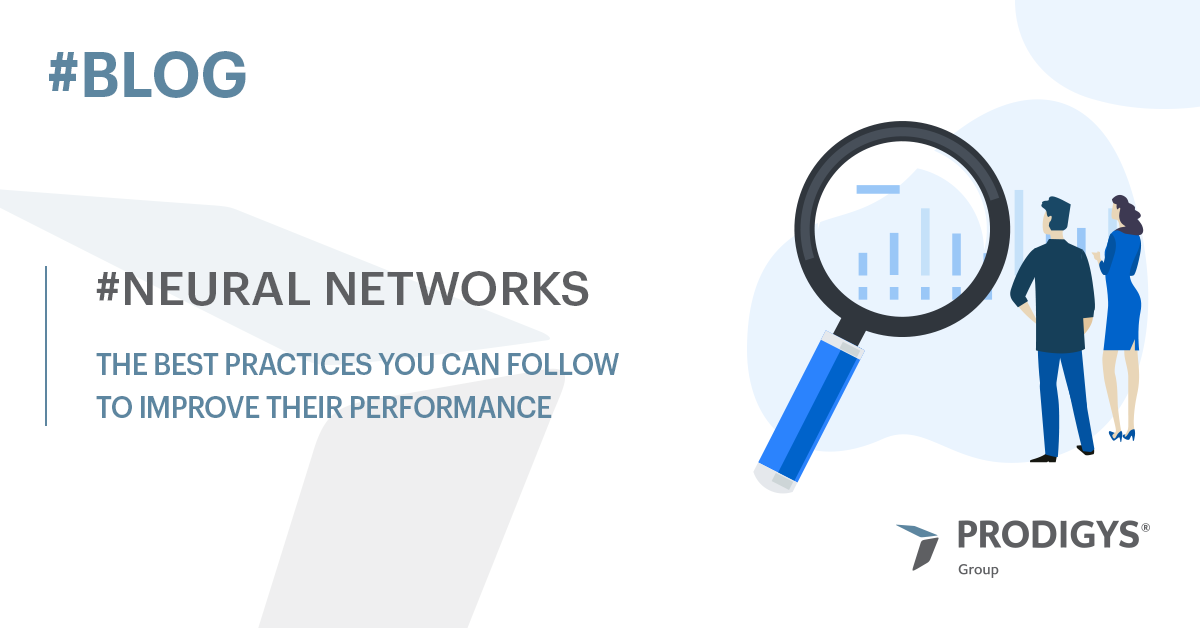# Good Practices for Neural Network

• On March 25, 2022

## Introduction

Today, we’ll see some of the most commonly used practices to improve neural networks. We will consider both actions you can perform on the dataset and on the network itself.

## Good Practices for the Dataset

Pre-processing

Almost always the initial dataset contains errors, missing or useless information, and other inconsistencies. By pre-processing your data, you can aim directly at fixing those issues. For instance, for missing data, you can use substitutes such as a zero or values calculated with averages and interpolations.

Data augmentation

If the dataset is not well-balanced or too small, you will need to add more data and data-augmentation techniques can help you with that. You can manipulate your initial dataset and create new sets that differ from the original. Knowing how to do this is key, but it varies depending on the context. For instance, if you consider the field of vehicle recognition, where you have a constant and repetitive pattern like that of the wheels on the road, a vertical flip that overturns the car would not create any new useful images for your scope.

## Good Practices for the Network

A neural network is made of an input layer, hidden layers and an output layer.

• In the input layer, you would ideally want the number of neurons to correspond to the number of features that the neural net will use to make predictions.
• For tabular data, you would want it to correspond to the main characteristics of the dataset.
• For images, you would want it o correspond to the size of the image, specifically the number of pixels (e.g., 16348 nodes for a 128x128px).
• The number of hidden layers would depend on the situation. It is recommended to start with 1-5 hidden layers and then start adding more. To choose the number of neurons, you would start by matching the number of layers and then decrease. Evidence shows that having a big first layer followed by smaller layers could lead to better performances.
• In the output layer, you would want to match the number of neurons to the number of predictions it needs to perform. This means that the number of neurons will be the same as the number of classifications.

### Activation functions

These functions should not work on negative ranges (this would otherwise compromise the following layers). They should be non-linear so that they cannot be grouped together as this would reduce the complexity of the network. Oversimplifying it would in fact compromise its efficiency.

### Loss functions

The loss function allows you to measure the accuracy of a statistical model to describe a dataset of empirical data of a particular phenomenon.

A model could correctly classify the various samples, but the loss function will show the difference between the predicted value and the real one. The lower the value, the better the model behaves.

This function, again, depends on the context. For a binary classification, binary_crossentropy would be best. For a multi-class, categorical cross_entropy would be better.

### Optimization algorithm

Optimization algorithms update the weights to reduce the loss function to a minimum. There are different types, but these are amongst the most famous: